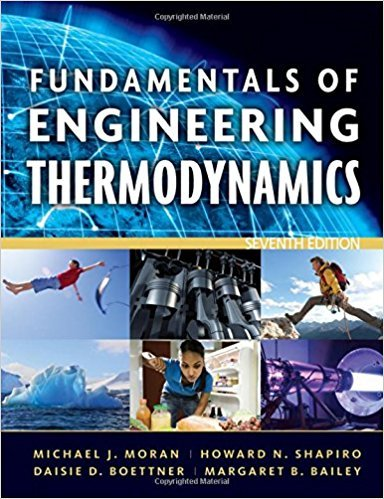×
Get Full Access to Fundamentals Of Engineering Thermodynamics - 7 Edition - Chapter 4 - Problem 22p
Get Full Access to Fundamentals Of Engineering Thermodynamics - 7 Edition - Chapter 4 - Problem 22p

×

# Applying Conservation of MassFigure P4.22 shows aISBN: 9780470495902 50

## Solution for problem 22P Chapter 4

Fundamentals of Engineering Thermodynamics | 7th Edition

• Textbook Solutions
• 2901 Step-by-step solutions solved by professors and subject experts
• Get 24/7 help from StudySoup virtual teaching assistantsFundamentals of Engineering Thermodynamics | 7th Edition

4 5 1 350 Reviews
13
1
Problem 22P

Problem 22P

Applying Conservation of Mass

Figure P4.22 shows a cylindrical tank being drained through a duct whose cross-sectional area is 3 × 10−4 m2. The velocity of the water at the exit varies according to (2gz)1/2, where z is the water level, in m, and g is the acceleration of gravity, 9.81 m/s2. The tank initially contains 2500 kg of liquid water. Taking the density of the water as 103 kg/m3, determine the time, in minutes, when the tank contains 900 kg of water.Fig. P4.22

Step-by-Step Solution:

Step 1 of  2

We have to determine the time when the tank contains 900 kg of water initially it contains 2500 kg of liquid water.

The mass rate balance for control volume is given by the expressionwhere ,is the mass flow rate at the exit

Now,cross sectional area = 3m2= velocity of water at the exit in m/sdensity of liquid at the exit

So,ButHence,Step 2 of 2

##### ISBN: 9780470495902

Unlock Textbook Solution